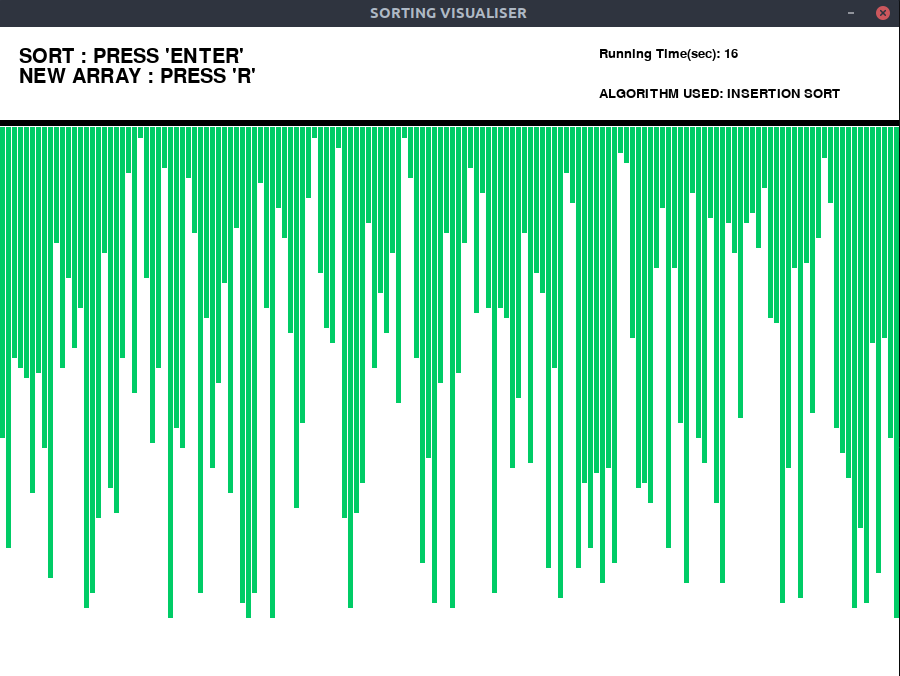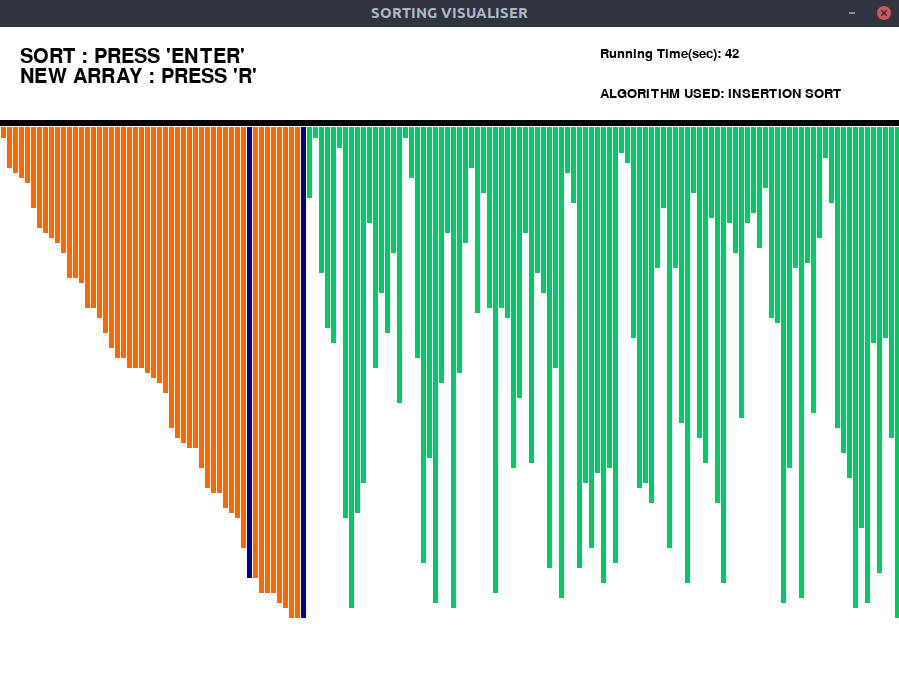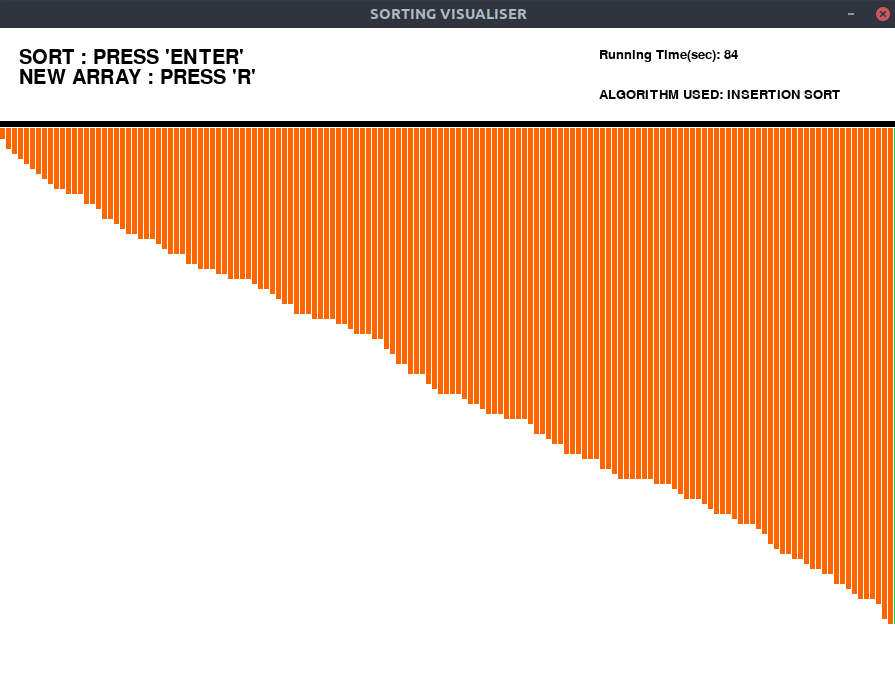# Sorting algorithm visualization : Insertion Sort

An algorithm like Insertion Sort can be understood easily by visualizing. In this article, a program that visualizes the Insertion Sort Algorithm has been implemented.
The Graphical User Interface(GUI) is implemented in python using pygame library.
Approach:

• Generate random array and fill the pygame window with bars. Bars are straight vertical lines, which represents array elements.
• Set all bars to green color (unsorted).
• Use pygame.time.delay() to slow down the algorithm, so that we can see the sorting process.
• Implement a timer to see how the algorithm performs.
• The actions are performed using ‘pygame.event.get()’ method, which stores all the events which user performs, such as start, reset.
• Blue color is used to highlight bars that are involved in sorting at a paticular time.
• Orange color highlights the bars sorted.

Observations:
We can clearly see from the Insertion Sort visualization, that insertion Sort is very slow compared to other sorting algorithms like Mergesort or Quicksort.
Examples:

Input:
Press “Enter” key to Perform Visualization.
Press “R” key to generate new array.
Output:
Initial:Sorting:Final:Please make sure to install the pygame library before running the below program.

Below is the implementation of the above visualizer:

## Python

 `# Python implementation of the  ` `# Sorting visualiser: Insertion Sort  ` ` `  `# Imports ` `import` `pygame ` `import` `random ` `import` `time ` `pygame.font.init() ` `startTime ``=` `time.time() ` `# Total window ` `screen ``=` `pygame.display.set_mode( ` `                        ``(``900``, ``650``) ` `                    ``) ` ` `  `# Title and Icon  ` `pygame.display.set_caption( ` `            ``"SORTING VISUALISER"` `            ``) ` ` `  `# Uncomment below lines for setting  ` `# up the icon for the visuliser ` `# img = pygame.image.load('sorticon.png') ` `# pygame.display.set_icon(img) ` ` `  `# Boolean variable to run  ` `# the program in while loop ` `run ``=` `True` ` `  `# Window size and some initials ` `width ``=` `900` `length ``=` `600` `array ``=``[``0``]``*``151` `arr_clr ``=``[(``0``, ``204``, ``102``)]``*``151` `clr_ind ``=` `0` `clr ``=``[(``0``, ``204``, ``102``), (``255``, ``0``, ``0``), \ ` `       ``(``0``, ``0``, ``153``), (``255``, ``102``, ``0``)] ` `fnt ``=` `pygame.font.SysFont(``"comicsans"``, ``30``) ` `fnt1 ``=` `pygame.font.SysFont(``"comicsans"``, ``20``) ` ` `  `# Function to generate new Array ` `def` `generate_arr(): ` `    ``for` `i ``in` `range``(``1``, ``151``): ` `        ``arr_clr[i]``=` `clr[``0``] ` `        ``array[i]``=` `random.randrange(``1``, ``100``) ` ` `  `# Initially generate a array ` `generate_arr()  ` ` `  `# Function to refill the  ` `# updates on the window  ` `def` `refill(): ` `    ``screen.fill((``255``, ``255``, ``255``)) ` `    ``draw() ` `    ``pygame.display.update() ` `    ``pygame.time.delay(``10``) ` ` `  `# Sorting Algorithm: Insertion sort ` `def` `insertionSort(array): ` ` `  `    ``for` `i ``in` `range``(``1``, ``len``(array)): ` `        ``pygame.event.pump()  ` `        ``refill() ` `        ``key ``=` `array[i] ` `        ``arr_clr[i]``=` `clr[``2``] ` `        ``j ``=` `i``-``1` `        ``while` `j>``=` `0` `and` `key

Output:

Attention reader! Don’t stop learning now. Get hold of all the important DSA concepts with the DSA Self Paced Course at a student-friendly price and become industry ready.

My Personal Notes arrow_drop_upCheck out this Author's contributed articles.

If you like GeeksforGeeks and would like to contribute, you can also write an article using contribute.geeksforgeeks.org or mail your article to contribute@geeksforgeeks.org. See your article appearing on the GeeksforGeeks main page and help other Geeks.

Please Improve this article if you find anything incorrect by clicking on the "Improve Article" button below.

Practice Tags :

Be the First to upvote.

Please write to us at contribute@geeksforgeeks.org to report any issue with the above content.